## ML Aggarwal Class 8 Solutions for ICSE Maths Chapter 2 Exponents and Powers Check Your Progress

Question 1.
Evaluate:Solution: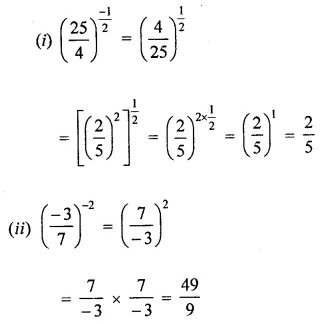Question 2.
Simplify: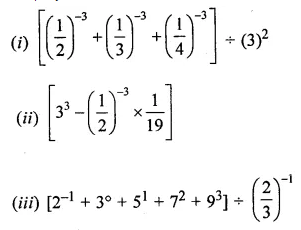Solution: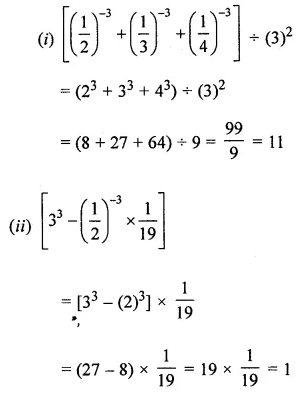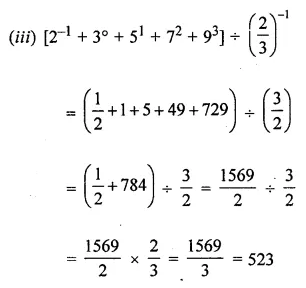Question 3.
Find the multiplicative inverse of $$\left(\frac{125}{27}\right)^{\frac{-2}{3}}$$
Solution: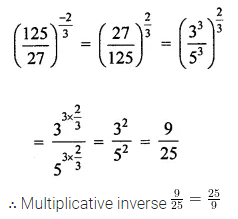Question 4.
Simplify and write in exponential form: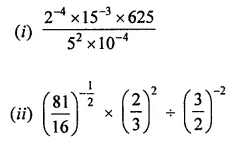Solution: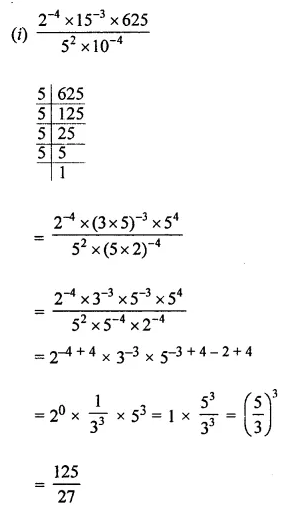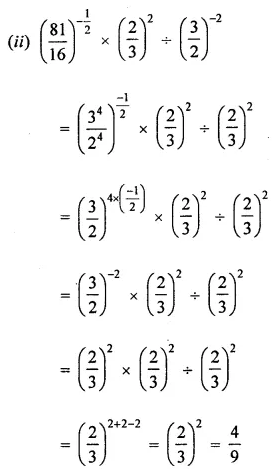Question 5.
Simplify: $$\frac{5^{n+2}-6+5^{n+1}}{13 \times 5^{n}-2 \times 5^{n+1}}$$
Solution:Question 6.
Prove that (a + b)-1 (a-1 + b-1) = (ab)-1.
Solution: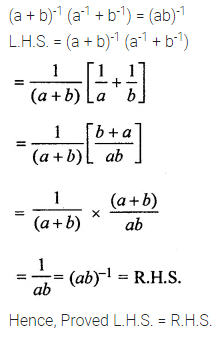Question 7.
Find the value of x for which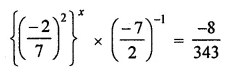Solution: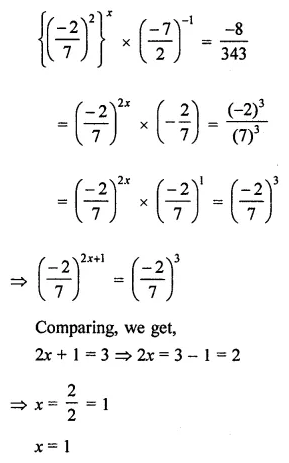Question 8.
If $$\frac{2^{-n} \times 8^{2 n+1} \times 16^{2 n}}{4^{3 n}}=\frac{1}{16}$$, find the value of n.
Solution: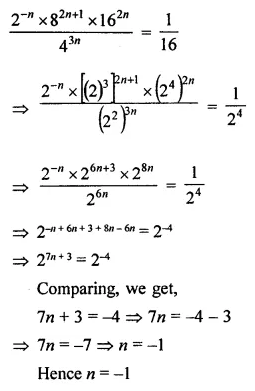Question 9.
By what number should $$\left[\left(\frac{-5}{2}\right)^{3}\right]^{-3}$$ be multiplied to get $$\left(\frac{-2}{5}\right)^{5}$$?
Solution: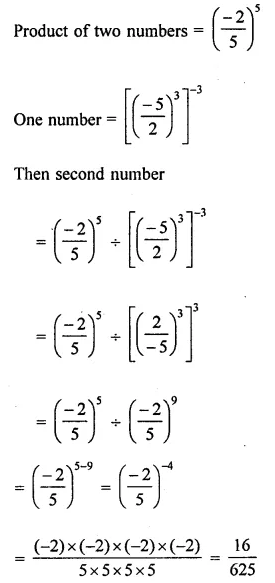Question 10.
By what number should $$\left(\frac{-3}{2}\right)^{-4}$$ be divided to get $$\left(\frac{-2}{3}\right)^{3}$$?
Solution: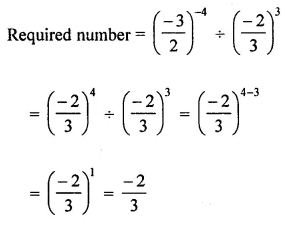Question 11.
Express the following numbers in standard form:
(i) 0.0000000003904
(ii) 12730000000000
Solution: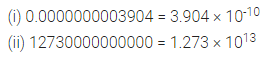Question 12.
Express the following numbers in the usual form:
(i) 5.73 × 10-11
(ii) 3.895 × 1015.
Solution: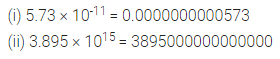Question 13.
If $$\left(\frac{9}{4}\right)^{-4} \times\left(\frac{2}{3}\right)^{3}=\left(\frac{p}{q}\right)^{11}$$, then find the value of $$\left(\frac{p}{q}\right)^{-2}$$.
Solution: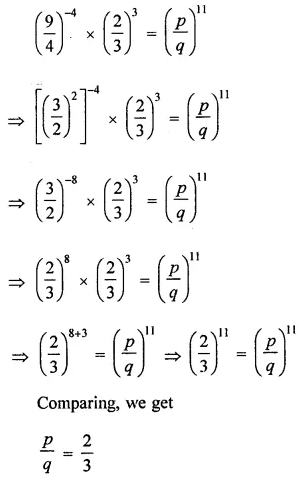Question 14.
Mass of the Earth is 5.97 × 1024 kg and mass of the Moon is 7.35 × 1022 kg. What is the difference of their masses?
Solution: